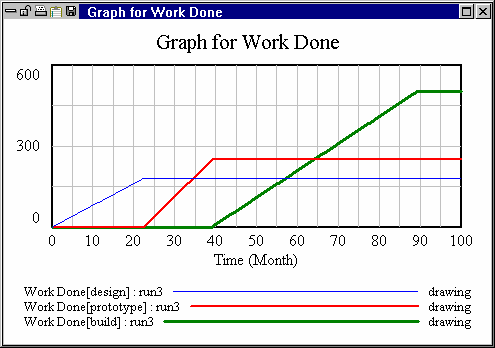# Vensim Help

 Normalized Lookups

The graphical function drawn above has the number of rabbits as its input and the number of rabbits that die per year as its output.  This is a difficult graph to create, and worse, it is very tricky to change.  Suppose, for example, you want to understand what happens when a longer lived breed of rabbits is introduced — the whole function needs to be redone.  Or suppose you want to understand the effects of increasing the carrying capacity of the rabbit's environment — the whole function again needs to be redone.

A normalized input is built around reference points such as 0,0 and 1,1.  The input is adjusted to be dimensionless and independent of the units of measure or scale of other variables in the model.  The output is very often dimensionless and is also independent of the units of measure and scale of other variables.  For example, suppose we were to measure Population in thousands of rabbits and deaths in thousands of rabbits per month.   A Lookup function taking Population as its input and having deaths as its output would no longer be valid.

On the other hand, a Lookup function, normalized by using Population relative to carrying capacityas its input and having the output effect on deaths act on a baseline or normal number of deaths (Population / average lifetime), does not need to be changed when units of measure or scale change.

Normalization allows us to achieve the desired behavioral relationship with a generalized set of numbers in the Lookup function.  If information about the size or characteristics of the population changes, you can simply change the value of carrying capacity or average lifetime, rather than the whole Lookup function.To normalize, divide the input variable by a normal or average value (e.g., Population / carrying capacity).  When the actual Population is equal to this normal value, the input (to the Lookup) is 1.  Other values of Population will vary the input either higher or lower than 1.  The output values of a Lookup are also usually made to vary about the value of 1.  The output of the Lookup can then be used to drive another model variable above or below its normal value.  Another way of saying this is:  when the input variable is equal to its normal or average value, the output from the Lookup is 1 and therefore has no effect on the connected output variable's value.

NOTE This formula is similar to the Lookup equation example in the beginning of this chapter y = fn (x), but takes two variables as the (normalized) input and computes the output relative to a normalized value : y = normal y * fn (x/normal x).